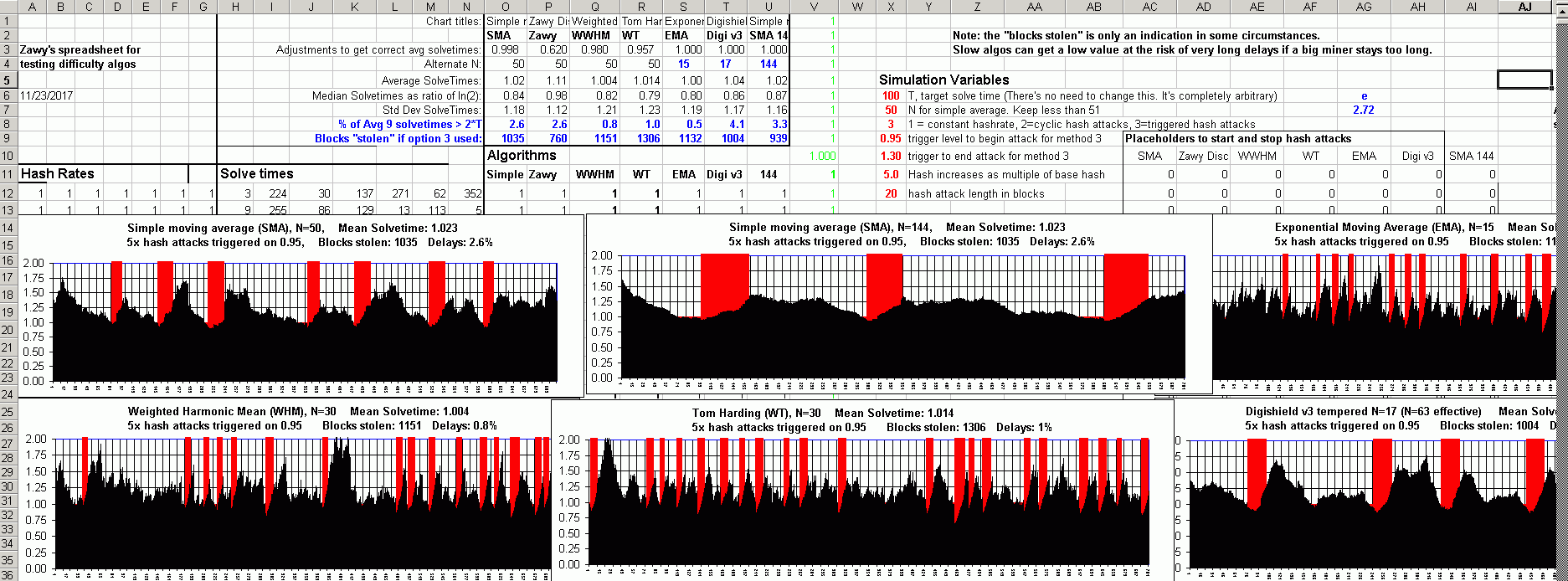# Best difficulty algorithm so far #28

Open
opened this issue Nov 20, 2017 · 3 comments

###zawy12 commented Nov 20, 2017 • edited

 I recommend one of the two following. They seem pretty much the same when the N of WWHM is 2x the N of EMA. In case I do not keep this page updated, I'll keep this blog post updated. ``````# Weighted-Weighted Harmonic Mean (WWHM) difficulty algorithm # Original idea from Tom Harding (Deger8) called "WT-144" # No limits, filtering, or tempering should be attempted. # MTP should not be used. # set constants N=60 T=600 # coin's Target solvetime. Value can be anything. adjust=0.99 # 0.98 for N=30, 0.99 for N=60 k = (N+1)/2 *adjust * T # algorithm previous_max=timestamp[height - N] for ( i = height-N+1; i < height+1; i++) { # (N most recent blocks) max_timestamp=max(timestamp[i], previous_max) solvetime = max_timestamp - previous_max solvetime=1 if solvetime < 1 solvetime = 10*T if solvetime > 10*T previous_max=max_timestamp j++ t += solvetime * j # give more weight to recent blocks d +=D[i] # sum the difficulties } # next i t=T*N if t < T*N # in case of startup weirdness keep t sane next_D = d * k / t # limits do not need to be used because of the above solvetime restrictions # but if you still want limits on D's change per block & expect max 5x hash rate # changes or want to replace the solvetime restrictions, use # limit = 5^(3/N) # next_D = previous_D*limit if next_D > previous_D*limit # next_D = previous_D/limit if next_D > previous_D/limit `````` ======================= ``````# Jacob Eliosoff's EMA (exponential moving average) # see https://en.wikipedia.org/wiki/Moving_average#Application_to_measuring_computer_performance # ST = previous solvetime # N=35 to 100 seems possible. Small N for small coins. N=70 for all might be best. # MTP should not be used ST = previous timestamp - timestamp before that ST = max(T/100,min(T*10, ST)) next_D = previous_D * ( T/ST + e^(-ST/T/N) * (1-T/ST) ) ``````###zawy12 commented Nov 28, 2017 • edited

 This is the new best one. It is beneficial over simple N=70 only if sudden hash changes are >3x. ``````# Dynamic EMA difficulty algo (Jacob Eliosoff's EMA and Zawy's adjustable window). # Bitcoin Cash dev (Amaury?) came up with the median of three to reduce timestamp errors. # For EMA origins see # https://en.wikipedia.org/wiki/Moving_average#Application_to_measuring_computer_performance # "Dynamic" means it triggers to a faster-responding value for N if a substantial change in hashrate # is detected. It increases from that event back to Nmax Nmax=70 # max EMA window Nmin=25 # min EMA window A=10, B=2, C=0.37 # A,B,C = 10,2,0.37 or 20, 1.65 0.45, # TS=timestamp, T=target solvetime, i.e. 600 seconds # Find the most recent unusual 20-block event for (i=height-Nmax to height) { # height=current block index if ( (median(TS[i],TS[i-1],TS[i-2]) - median(TS[i-20],TS[i-21],TS[i-22]))/T/A > B or (median(TS[i],TS[i-1],TS[i-2]) - median(TS[i-20],TS[i-21],TS[i-22]))/T/A < C ) { unusual_event=height - i + Nmin } } N = min(Nmax, unusual_event)) # now use the EMA difficulty algorithm with this N `````` Here are some comparisons under sudden hashrate changes and constant hashrate.

###zawy12 commented Nov 29, 2017

 I corrected a code error in the previous post.
added a commit to thaerkh/monero that referenced this issue Dec 5, 2017
``` Add WHM difficulty adjustment algorithm. ```
``` cdc50a3 ```
```Algorithm design by @zawy12 kyuupichan/difficulty#28

- rolling window N=60
- Block future limit of 20 minutes
- Timestamp check window of 12 blocks```
added a commit to thaerkh/monero that referenced this issue Dec 6, 2017
``` Add WHM difficulty adjustment algorithm. ```
``` 9a80015 ```
```Algorithm design by @zawy12 kyuupichan/difficulty#28

- rolling window N=60
- Block future limit of 20 minutes
- Timestamp check window of 12 blocks```
added a commit to thaerkh/monero that referenced this issue Dec 6, 2017
``` Add WHM difficulty adjustment algorithm. ```
``` 1d706e0 ```
```Algorithm design by @zawy12 kyuupichan/difficulty#28

- rolling window N=60
- Block future limit of 20 minutes
- Timestamp check window of 12 blocks```
added a commit to thaerkh/monero that referenced this issue Dec 6, 2017
``` Add WHM difficulty adjustment algorithm. ```
``` ecfe57f ```
```Algorithm design by @zawy12 kyuupichan/difficulty#28